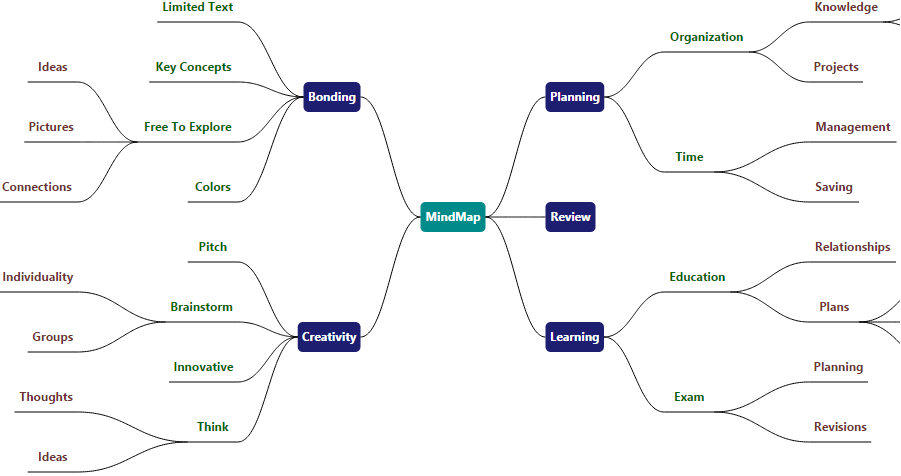# Exporting

28 Jun 20173 minutes to read

Diagram provides support to export its content as image/svg files.
The client side method `exportDiagram` helps to export the Diagram. The following code illustrates how to export the Diagram as image.

• JAVASCRIPT
• ``````var diagram = \$("#diagram").ejDiagram("instance");
//Exports the Diagram as an image of JPEG format
diagram.exportDiagram();``````## Exporting options

Diagram provides support to export the desired region of the Diagram to desired formats.

### FileName

FileName is the name of the file to be downloaded. By default, the file name is set as “Diagram”.

### Format

Format is to specify the type/format of the exported file. By default, Diagram is exported as .jpg format. You can export Diagram to the following formats.

• JPG
• PNG
• BMP
• SVG

### Margin

Margin specifies the amount of space that has to be left around the Diagram.

• JAVASCRIPT
• ``````var diagram = \$("#diagram").ejDiagram("instance");
var options = {
fileName: "diagram",
//Margin to the exported file/data
margin: {
left: 30,
right: 30,
top: 30,
bottom: 30
}
};
diagram.exportDiagram(options);``````

### Mode

Mode specifies whether the Diagram is to be exported as files or as data(ImageURL/SVG). The exporting options are as follows.

• Exports Diagram as data of formats ImageURL/SVG

The following code example illustrates how to export the Diagram as raw data.

• JAVASCRIPT
• ``````var diagram = \$("#diagram").ejDiagram("instance");
var options = {
fileName: "diagram",
//Specifies whether to export as files/data
mode: "data"
};
diagram.exportDiagram(options);``````

NOTE

In IE-9, Diagrams cannot be exported as downloadable files. Instead, Diagram provides the exported data (DataURL/SVG) that enables to export it from application.

### Region

You can export any particular region of the Diagram and the region is categorized as follows.

• Region that fits all nodes and connectors that are added to model
• Region that fits all pages (single or multiple pages based on page settings)

The following code example illustrates how to export the region occupied by the Diagram elements.

• JAVASCRIPT
• ``````var diagram = \$("#diagram").ejDiagram("instance");
var options = {
//Specifies the exporting region
mode: "content"
};
diagram.exportDiagram(options);``````

The following code example illustrates how to export any specific region of the Diagram.

• JAVASCRIPT
• ``````var diagram = \$("#diagram").ejDiagram("instance");
var options = {
fileName: "diagram",
//Specifies whether to export as files/data